# Fraction calculator

The calculator performs basic and advanced operations with fractions, expressions with fractions combined with integers, decimals, and mixed numbers. It also shows detailed step-by-step information about the fraction calculation procedure. Solve problems with two, three, or more fractions and numbers in one expression.

## Result:

### 21/4 - 11/5 = 21/20 = 1 1/20 = 1.05

Spelled result in words is twenty-one twentieths (or one and one twentieth).

### How do you solve fractions step by step?

1. Conversion a mixed number 2 1/4 to a improper fraction: 2 1/4 = 2 1/4 = 2 · 4 + 1/4 = 8 + 1/4 = 9/4

To find a new numerator:
a) Multiply the whole number 2 by the denominator 4. Whole number 2 equally 2 * 4/4 = 8/4
b) Add the answer from previous step 8 to the numerator 1. New numerator is 8 + 1 = 9
c) Write a previous answer (new numerator 9) over the denominator 4.

Two and one quarter is nine quarters
2. Conversion a mixed number 1 1/5 to a improper fraction: 1 1/5 = 1 1/5 = 1 · 5 + 1/5 = 5 + 1/5 = 6/5

To find a new numerator:
a) Multiply the whole number 1 by the denominator 5. Whole number 1 equally 1 * 5/5 = 5/5
b) Add the answer from previous step 5 to the numerator 1. New numerator is 5 + 1 = 6
c) Write a previous answer (new numerator 6) over the denominator 5.

One and one fifth is six fifths
3. Subtract: 9/4 - 6/5 = 9 · 5/4 · 5 - 6 · 4/5 · 4 = 45/20 - 24/20 = 45 - 24/20 = 21/20
For adding, subtracting, and comparing fractions, it is suitable to adjust both fractions to a common (equal, identical) denominator. The common denominator you can calculate as the least common multiple of both denominators - LCM(4, 5) = 20. In practice, it is enough to find the common denominator (not necessarily the lowest) by multiplying the denominators: 4 × 5 = 20. In the following intermediate step, the fraction result cannot be further simplified by canceling.
In other words - nine quarters minus six fifths = twenty-one twentieths.

#### Rules for expressions with fractions:

Fractions - simply use a forward slash between the numerator and denominator, i.e., for five-hundredths, enter 5/100. If you are using mixed numbers, be sure to leave a single space between the whole and fraction part.
The slash separates the numerator (number above a fraction line) and denominator (number below).

Mixed numerals (mixed fractions or mixed numbers) write as integer separated by one space and fraction i.e., 1 2/3 (having the same sign). An example of a negative mixed fraction: -5 1/2.
Because slash is both signs for fraction line and division, we recommended use colon (:) as the operator of division fractions i.e., 1/2 : 3.

Decimals (decimal numbers) enter with a decimal point . and they are automatically converted to fractions - i.e. 1.45.

The colon : and slash / is the symbol of division. Can be used to divide mixed numbers 1 2/3 : 4 3/8 or can be used for write complex fractions i.e. 1/2 : 1/3.
An asterisk * or × is the symbol for multiplication.
Plus + is addition, minus sign - is subtraction and ()[] is mathematical parentheses.
The exponentiation/power symbol is ^ - for example: (7/8-4/5)^2 = (7/8-4/5)2

#### Examples:

subtracting fractions: 2/3 - 1/2
multiplying fractions: 7/8 * 3/9
dividing Fractions: 1/2 : 3/4
exponentiation of fraction: 3/5^3
fractional exponents: 16 ^ 1/2
adding fractions and mixed numbers: 8/5 + 6 2/7
dividing integer and fraction: 5 ÷ 1/2
complex fractions: 5/8 : 2 2/3
decimal to fraction: 0.625
Fraction to Decimal: 1/4
Fraction to Percent: 1/8 %
comparing fractions: 1/4 2/3
multiplying a fraction by a whole number: 6 * 3/4
square root of a fraction: sqrt(1/16)
reducing or simplifying the fraction (simplification) - dividing the numerator and denominator of a fraction by the same non-zero number - equivalent fraction: 4/22
expression with brackets: 1/3 * (1/2 - 3 3/8)
compound fraction: 3/4 of 5/7
fractions multiple: 2/3 of 3/5
divide to find the quotient: 3/5 ÷ 2/3

The calculator follows well-known rules for order of operations. The most common mnemonics for remembering this order of operations are:
PEMDAS - Parentheses, Exponents, Multiplication, Division, Addition, Subtraction.
BEDMAS - Brackets, Exponents, Division, Multiplication, Addition, Subtraction
BODMAS - Brackets, Of or Order, Division, Multiplication, Addition, Subtraction.
GEMDAS - Grouping Symbols - brackets (){}, Exponents, Multiplication, Division, Addition, Subtraction.
Be careful, always do multiplication and division before addition and subtraction. Some operators (+ and -) and (* and /) has the same priority and then must evaluate from left to right.

## Fractions in word problems:

• Square metal sheetWe cut out four squares of 300 mm side from a square sheet metal plate with a side of 0,7 m. Express the fraction and the percentage of waste from the square metal sheet.
• Fractions and mixed numerals(a) Convert the following mixed numbers to improper fractions. i. 3 5/8 ii. 7 7/6 (b) Convert the following improper fraction to a mixed number. i. 13/4 ii. 78/5 (c) Simplify these fractions to their lowest terms. i. 36/42 ii. 27/45 2. evaluate the follow
• PoundsThree pounds subtract 1/3 of a pound.
• Cherries 2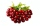If a farmer reaped 636 cherries and he sold one third to a shop keeper, how many did he retain?
• There 17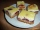There is 3/4 of a cake on a plate in Maria's kitchen.  Silvia sees the cake and eats 1/5 of the cake.  Then Franca takes 1/3 of what was there and shares half of her portion with Antonella.  What fraction of the cake is left?
• Jose studiedJose studied for 4 and 1/2 hours on Saturday and another 6 and 1/4 hours on Sunday. How many subjects did he study if he has alloted 1 and 1/2 hours per subject on Saturday and 1 and 1/4 hours per subject on Sunday?
• Ali bought 2Ali bought 5/6 litre of milk. He drank 1/2 litre and his brother drank 1/6 litre. How much litre of milk left?
• Hotel 4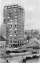A 360 room hotel has 1/3 of its rooms occupied at present. How many rooms are empty?
• Bucket of clay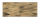Tina and Bill share a 12-ounce bucket of clay. By the end of the week, Tina has used 1/6 of the bucket, and Bill has used 2/3 of the bucket of clay. How many ounces are left in the bucket?
• Mountain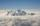Mountain has an elevation of 7450 meters and in the morning is the middle portion thereof in the clouds. How many meters of height is in the sky if below the clouds are 2,000 meters, and above clouds are two-fifths of the mountain's elevation?
• Mrs. SusanMrs. Susan bought 1/8 m of curtain cloth. She used 3/5 m to make a curtain for the living room window. How many meters of cloth were not used?
• Translate 2Translate the given phrases to mathematical phrases. Thrice the sum of three fifths and two thirds less one half is what number?
• Mixed numbers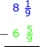Five and two-thirds minus 2 and one-half equals what number? A three and one-sixth B three and two-thirds C three and one-half D three and five-sixths